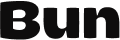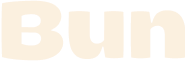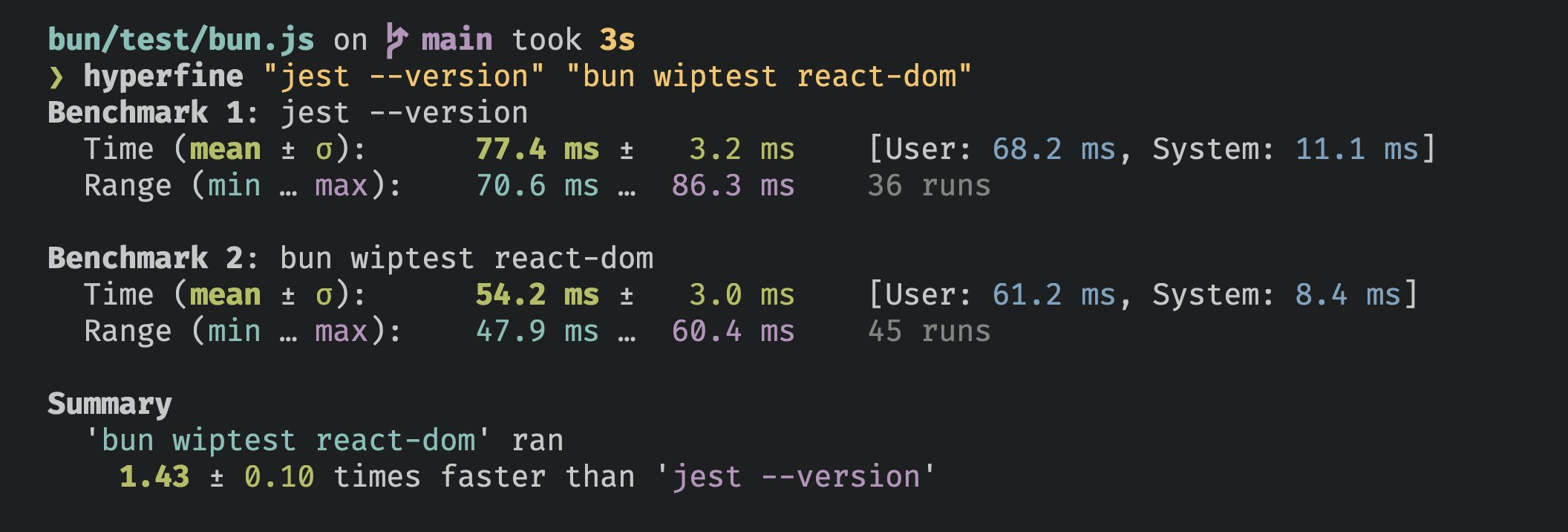# `bun test`

Bun ships with a built-in test runner.

``bun test``

Tests are written in JavaScript or TypeScript with a Jest-like API.

math.test.ts
``````import { expect, test } from "bun:test";

test("2 + 2", () => {
expect(2 + 2).toBe(4);
});
``````

It's fast.Bun runs 266 React SSR tests faster than Jest can print its version number.

The runner recursively searches the working directory for files that match the following patterns:

• `*.test.{js|jsx|ts|tsx}`
• `*_test.{js|jsx|ts|tsx}`
• `*.spec.{js|jsx|ts|tsx}`
• `*_spec.{js|jsx|ts|tsx}`

You can filter the set of tests to run by passing additional positional arguments to `bun test`. Any file in the directory with an absolute path that contains one of the filters will run. Commonly, these filters will be file or directory names; glob patterns are not yet supported.

``bun test <filter> <filter> ...``

## Writing tests

Define tests with a Jest-like API imported from the built-in `bun:test` module.

math.test.ts
``````import { expect, test } from "bun:test";

test("2 + 2", () => {
expect(2 + 2).toBe(4);
});
``````

Group tests into suites with `describe`.

math.test.ts
``````import { expect, test, describe } from "bun:test";

describe("arithmetic", () => {
test("2 + 2", () => {
expect(2 + 2).toBe(4);
});

test("2 * 2", () => {
expect(2 * 2).toBe(4);
});
});
``````

Tests can be `async`.

``````import { expect, test } from "bun:test";

test("2 * 2", async () => {
const result = await Promise.resolve(2 * 2);
expect(result).toEqual(4);
});
``````

Alternatively, use the `done` callback to signal completion. If you include the `done` callback as a parameter in your test definition, you must call it or the test will hang.

``````import { expect, test } from "bun:test";

test("2 * 2", done => {
Promise.resolve(2 * 2).then(result => {
expect(result).toEqual(4);
done();
});
});
``````

Perform per-test setup and teardown logic with `beforeEach` and `afterEach`.

``````import { expect, test } from "bun:test";

beforeEach(() => {
console.log("running test.");
});

afterEach(() => {
console.log("done with test.");
});

// tests...
``````

Perform per-scope setup and teardown logic with `beforeAll` and `afterAll`. At the top-level, the scope is the current file; in a `describe` block, the scope is the block itself.

``````import { expect, test, beforeAll, afterAll } from "bun:test";

let db: Database;
beforeAll(() => {
// connect to database
});

afterAll(() => {
// close connection
});

// tests...
``````

Skip individual tests with `test.skip`.

``````import { expect, test } from "bun:test";

test.skip("wat", () => {
// TODO: fix this
expect(0.1 + 0.2).toEqual(0.3);
});
``````

## Expect matchers

Bun implements the following matchers. Full Jest compatibility is on the roadmap; track progress here.